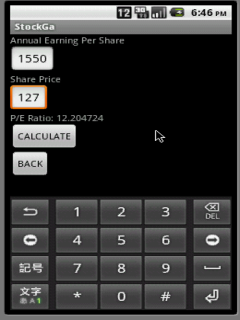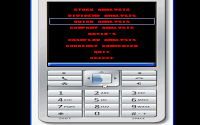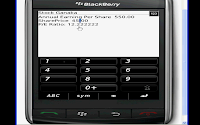Showing posts with label Stock. Show all posts
Showing posts with label Stock. Show all posts

### StockGa

Everyone wants to know the return on their investment in stock market. While the short term movements may be determined by market environment, long-term trends depend primarily on company financial health and performance. So how do you deduce the financial health of the company from the data company gives. Here StockGa comes into picture. It gives necessary tools to calculate the company financial health and performance.

STOCKGA 2.2
StockGa is Android based calculator for calculating Stock and Equity parameters of the company, which help in knowing the value of the stock. You are no longer are required to remember the formulas, carry a pen, pencil or a calculator to endlessly type and write the amounts. This calculator provides the necessary values in touch of a button. You can use StockGa to calculate the following. This calculator seamlessly works on the Android mobiles. The calculator does the computation of the following calculations provided below. Has user friendly operation. Simple key operation and also Touch screen interaction. It Calculates

Stock Analysis
Stock price
Expected Return
Holding value
Change in Holding value
Book to Market Ratio
Return on Equity

Dividend Analysis
Dividend per Share
Earning per share
P/E Ratio
Payout RatioNet Margin
Return of Equity
Cash Flow

Quick Analysis
PE Ratio
Price to sales Ratio
Return of Equity
PEG Ratio
Debt to Asset Ratio
Book to Market Ratio

Cash Flow Analysis
Free Cash Flow
Free Cash Flow to Equity
Price to Cash Flow Ratio
Free Cash Flow to Firm
Operating Cash Flow
Return on Assets
Operating Cash Flow Ratio

Activity Ratios
Stock Turnover Ratio
Debtors Turnover Ratio
Average Collection Period
Creditors Turnover Ratio
Working Capital Turnover Ratio
Fixed Assets Turnover Ratio

Leverage Ratio's
Debt to Equity Ratio
Equity Ratio
Fixed Asset to Equity Ratio
Current Assets to Equity Ratio
Debt Service Ratio
Capital Gearing RatioLiquidity and Efficiency
Current Ratio
Quick Ratio
Receivables Turnover Ratio
Average Age of Receivables
Stock Turnover Ratio
Number of Days for Inventory Turnover

Profitability Ratio's
Gross profit Ratio
Net Profit Ratio
Net Worth
Return of Capital Expenditure
Expense Ratio
Dividend Yield Ratio
Operating Ratio

Book Value
Price / Book Value Ratio
Tangible Book Value
Profit Margin
Debt to Cost Ratio
PEG Ratio
Enterprise value
Enterprise value Multiple

Capital
Working Capital
Current Ratio
Quick Ratio
Debt to Equity Ratio
Working Capital per Dollar sales
Working Capital Turnover Ratio
Debt Coverage.

Supporting phones
Android Ver.1.6 and Above

### StockGanaka

Everyone wants to know the return on their investment in stock market. While the short term movements may be determined by market environment, long-term trends depend primarily on company financial health and performance. So how do you deduce the financial health of the company from the data company gives.  Here StockGanaka comes into picture. It gives necessary tools to calculate the company financial health and performance.

STOCKGANAKAStockGanaka is mobile based calculator for calculating parameters of the company, which help in knowing the value of the stock. You are no longer are required to remember the formulas, carry a pen, pencil or a calculator to endlessly type and write the amounts. This calculator provides the necessary values in touch of a button. You can use StockGanaka to calculate the following.

StockGanaka 1.1
This calculator seamlessly works most of the mobiles having java and also Blackberry. The calculator does the computation of the following calculations provided below. Has user friendly operation. Simple key operation and also Touch screen interaction. It Calculates

Stock Analysis
Stock price
Expected Return
Holding value
Change in Holding value
Book to Market Ratio
Return on Equity

Dividend Analysis
Dividend per Share
Earning per share
P/E Ratio
Payout Ratio
Net Margin
Return of Equity
Cash FlowQuick Analysis
PE Ratio
Price to sales Ratio
Return of Equity
PEG Ratio
Debt to Asset Ratio
Book to Market Ratio

Cash Flow Analysis
Free Cash Flow
Free Cash Flow to Equity
Price to Cash Flow Ratio
Free Cash Flow to Firm
Operating Cash Flow
Return on Assets
Operating Cash Flow Ratio

Activity Ratios
Stock Turnover Ratio
Debtors Turnover Ratio
Average Collection Period
Creditors Turnover Ratio
Working Capital Turnover Ratio
Fixed Assets Turnover Ratio

Leverage Ratio's
Debt to Equity Ratio
Equity Ratio
Fixed Asset to Equity Ratio
Current Assets to Equity Ratio
Debt Service Ratio
Capital Gearing Ratio

Liquidity and Efficiency
Current Ratio
Quick Ratio
Receivables Turnover Ratio
Average Age of Receivables
Stock Turnover Ratio
Number of Days for Inventory TurnoverProfitability Ratio's
Gross profit Ratio
Net Profit Ratio
Net Worth
Return of Capital Expenditure
Expense Ratio
Dividend Yield Ratio
Operating Ratio

Book Value
Price / Book Value Ratio
Tangible Book Value
Profit Margin
Debt to Cost Ratio
PEG Ratio
Enterprise value
Enterprise value Multiple

Capital
Working Capital
Current Ratio
Quick Ratio
Debt to Equity Ratio
Working Capital per Dollar sales
Working Capital Turnover Ratio
Debt Coverage.Key-control
Up and Down Arrow keys to Browse Menu. Select or center key to select the Option. Touch screen choose the option and choose select. On the Parameter screen , you enter the data and select calculate. choose exit to get-back to menu. select quit to quit application.

Supporting phones
All Java Enabled phone and Blackberry phones.